How Cheenta works to ensure student success?
Explore the Back-Story

# Test of Mathematics Solution Subjective 33 - Symmetrical Minima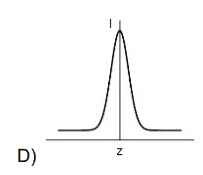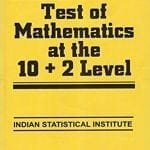Test of Mathematics Solution Subjective 33 (from ISI Entrance). The book, Test of Mathematics at 10+2 Level is Published by East West Press. This problem book is indispensable for the preparation of I.S.I. B.Stat and B.Math Entrance.

## Problem

Let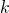be a fixed odd positive integer. Find the minimum value of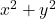, where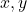are non-negative integers and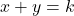.

## Solution

We have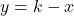. Therefore we get an equation in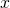whereis a constant, precisely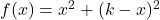.

To minimise, we differentiate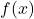w.r.t.

So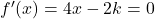(for minimum)

That gives us.

But the question tell us thatis odd andis an integer. therefore we have to take the closest possible integer value to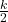, which is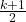and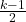.

As already defined, takingto be any one of the above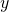automatically takes the other value.

Therefore the minimum value ofis given by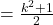.Test of Mathematics Solution Subjective 33 (from ISI Entrance). The book, Test of Mathematics at 10+2 Level is Published by East West Press. This problem book is indispensable for the preparation of I.S.I. B.Stat and B.Math Entrance.

## Problem

Letbe a fixed odd positive integer. Find the minimum value of, whereare non-negative integers and.

## Solution

We have. Therefore we get an equation inwhereis a constant, precisely.

To minimise, we differentiatew.r.t.

So(for minimum)

That gives us.

But the question tell us thatis odd andis an integer. therefore we have to take the closest possible integer value to, which isand.

As already defined, takingto be any one of the aboveautomatically takes the other value.

Therefore the minimum value ofis given by.

### Knowledge Partner